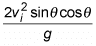# Finding range of a projectile from a graph

Hey,
I am writing a report where I am trying to investigate the effect of initial velocity on the range of a projectile. Does anyone know any graphs I could use to help me find the range?
Thanks,

## Answers and Replies

Prateek Kumar JainRange of the Projectile fired at an angle θ from the ground with an initial velocity Vi is given bySo Range is proportional to square of initial velocity keeping angle of projection θ as constant.

But I don't understand your question. Which graph you are talking about?

View attachment 102175

Range of the Projectile fired at an angle θ from the ground with an initial velocity Vi is given by

View attachment 102176

So Range is proportional to square of initial velocity keeping angle of projection θ as constant.

But I don't understand your question. Which graph you are talking about?

Apologies, so the report is being done with a sling shot, where the rubberband will be extended to different lengths, as this will affect the initial velocity. Angle is being kept constant and I am not sure which graph to use, however I am expected to manipulate a graph to show the relationship between initial velocity and range, and was wondering if it was possible to find the range through the gradient of a graph.

tommyxu3
and was wondering if it was possible to find the range through the gradient of a graph
What do you mean of the "gradient" of the graph? Your graph must mean the range ##R## versus the initial velocity ##v,## and in this single-variable function, the gradient is just the slope.

What do you mean of the "gradient" of the graph? Your graph must mean the range ##R## versus the initial velocity ##v,## and in this single-variable function, the gradient is just the slope.
ok yeah I just meant the slope when I said gradient, and was wondering if there were any graphs where the slope is equal to the range, but if not I will just do a graph that shows the range vs initial velocity,
Thanks,

tommyxu3
the slope is equal to the range
If you knew the result ##R=\frac{v^2\sin 2\theta}{g},## then you can easily get the slope ##R'(v)=\frac{2v\sin 2\theta}{g}## and can get what point meets your demand, which, however, the condition seems not meaningful... or?